Mathematics

# Prime Numbers

91 views

 1 Introduction 2 What are Prime Numbers? 3 List/Chart/History(Background) of Prime Numbers 4 Twin-Primes and Co-Primes 5 Sieve of Eratosthenes 6 FAQs

09 October 2020

## Introduction

This article endeavors to provide a ground and even a bridge between theory and experiment in the matter of prime numbers. Prime numbers belong to an exclusive world of intellectual conceptions. Prime Numbers enjoy simple, elegant description, yet complex in detail. The article tasks to find, characterize and apply the primes in various domains.

It is a straight forward math concept and as you progress through your mathematical career, you will see that there are actually fairly sophisticated concepts that can be built on the top of the idea of prime numbers.

## What are Prime Numbers?

A prime number is a positive integer (let us denote the prime number as p for further reference) having exactly two positive divisors, namely 1 and p itself.

In other words, a number is defined as a prime number, if it is a natural number (1 2 3… that is positive integers) divisible by exactly two different natural numbers. One of those numbers is 1 and the other one is the number itself. These are the two numbers it is divisible by.

Another form to define a prime number is that it cannot be broken further into a product of two natural numbers other than 1 and itself.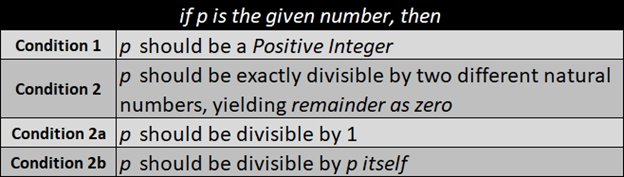Remember to use the Conditions

Condition 1
p should be a Positive Integer

Condition 2
p should be exactly divisible by two different natural numbers, yielding remainder as zero

Condition 2a
p should be divisible by 1

Condition 2b
p should be divisible by p itself

Make a checklist as shown below, to ease figuring out the conditions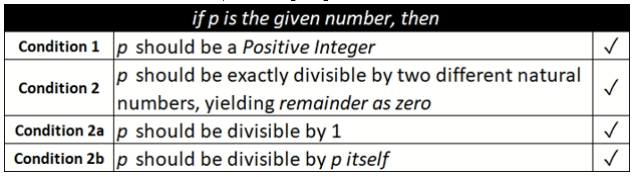### Case 1

Consider number 1

Verify the numbers with given conditions,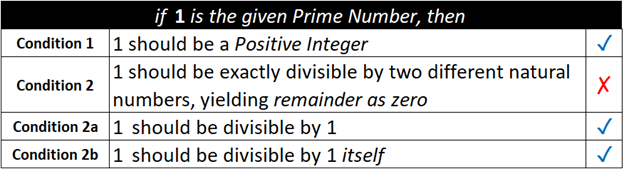Hence the number 1, is not a prime number

### Case 2

Consider number 2

Verify the numbers with given conditions,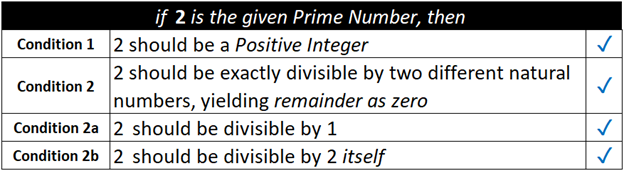Hence the number 2, is a prime number

### Case 3

Consider number 3

Verify the numbers with given conditions,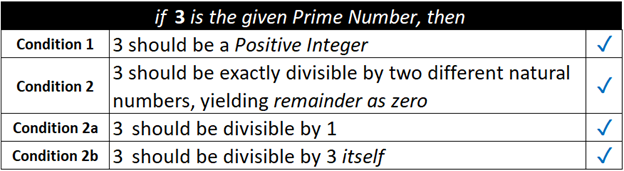Hence the number 3, is a prime number

### Case 4

Consider number 4

Verify the numbers with given conditions,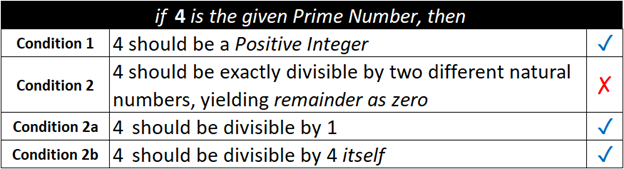4 is divisible by not just 1 and 4, but also by 2.

In other words, 4 can be broken as a product of two natural numbers, 2 x 2.

Hence the number 4, is not a prime number

### Case 5

Consider number 5

Verify the numbers with given conditions,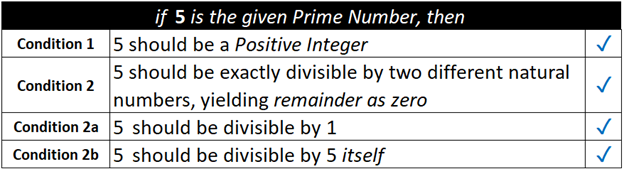Hence the number 5, is a prime number

### Case 6

Consider number 6

Verify the numbers with given conditions,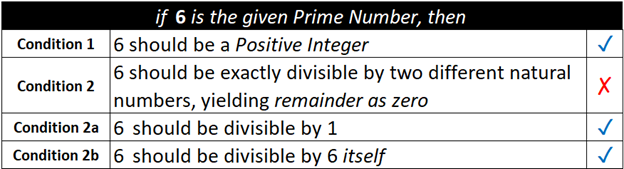6 is divisible by not just 1 and 6, but also by 2 and 3.

In other words, 6 can be broken as a product of natural numbers, 2 x 3 and 3 x 2.

Hence the number 6, is not a prime number

### Case 7

Consider number 7

Verify the numbers with given conditions,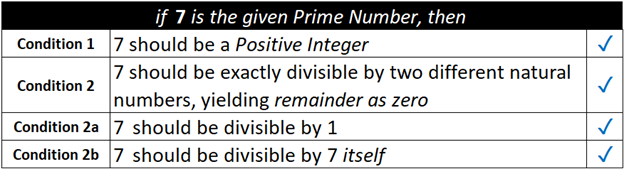Hence the number 7, is a prime number

Prime Numbers are kind of building blocks of numbers

Cannot break them down anymore.

Cannot break them into products of smaller natural numbers

Example in case 7. The number 7 cannot be broken down anymore,

in other words, 7 = seven times 1 or 1 time seven (1 x 7 or 7 x 1)

in this case, the number 7 could not be broken down further. It is noticed that 7 exists there again as seven times 1 or 1 time seven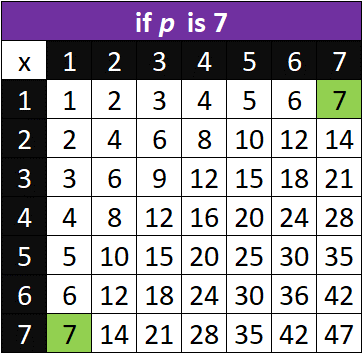This is the beauty of Prime Numbers

On the contrary lets us consider Case 4 and 6 which are not Prime in nature

Case 4. The number 4 can be broken down further, as a product of 2 x 2 apart from 1 x 4 and 4 x 1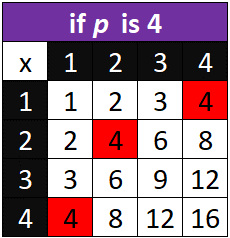Case 6. The number 6 can be broken down further, as a product of 2 x 3 apart from 1 x 6 and 6 x 1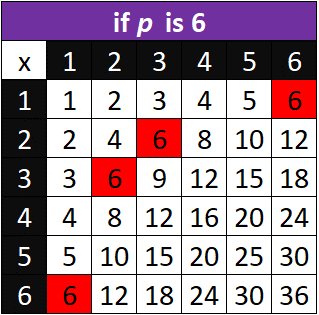In short, Prime Numbers cannot be drilled down.

Let us work with larger numbers

### Case 8

Consider number 16

Verify the numbers with given conditions,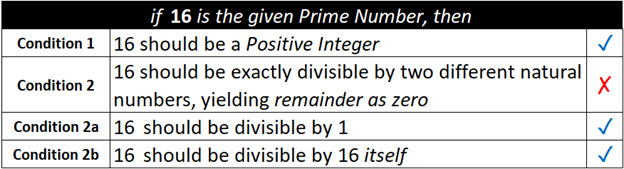16 is divisible by not just 1 and 16, but also by 2, 4, and 8.

In other words, 16 can be broken as a product of natural numbers, 2 x 8, 4 x 4, 8 x 2.

Hence the number 16, is not a prime number

### Case 9

Consider number 17

Verify the numbers with given conditions,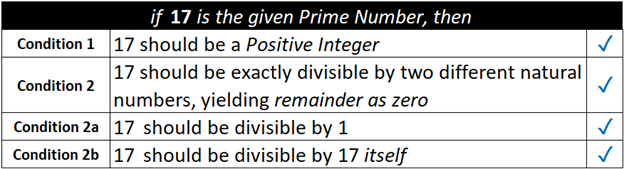Hence the number 17, is a prime number

### Case 10

Consider number 51

Verify the numbers with given conditions,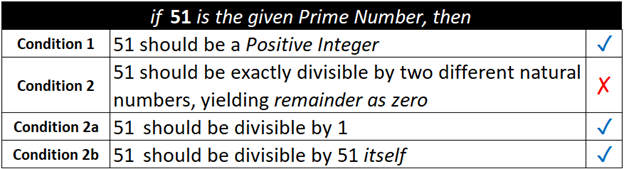51 is divisible by not just 1 and 51, but also by 3.

In other words, 51 can be broken as a product of natural numbers, 3 x 17 and 17 x 3.

Hence the number 51, is not a prime number

## List/Chart/History(Background) of Prime Numbers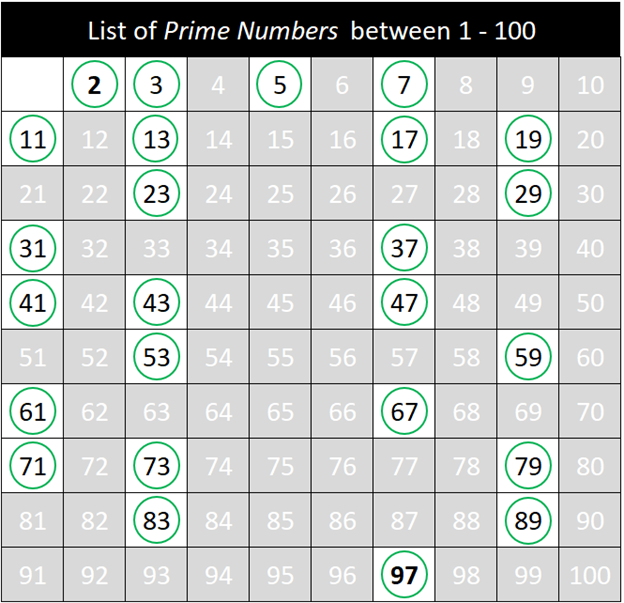Ancient Greek mathematicians were the first to extensively study Prime Numbers and their properties. The mathematicians of Pythagoras's school (500 BC to 300 BC) were interested in Prime Numbers for their mystical and numerological properties. They understood the idea of primality and were interested in perfect and amicable numbers.

In 300 BC, when Euclid's Elements appeared, several important results about Prime Numbers were proved. Euclid proof of the Fundamental Theorem of Arithmetic explains that “Every integer can be written as a product of Prime Numbers in an essentially unique way”.

In 200 BC, the Greek Eratosthenes devised an algorithm to calculate Prime Numbers, which in modern days is called the Sieve of Eratosthenes.

During the 17th Century, the next important developments in Prime Numbers were made by Fermat. Fermat's Little Theorem is the basis for many other results in Number Theory and is the basis for methods of checking whether numbers are prime which are still in use on today's electronic computers.

In 1952 the Mersenne numbers were proved to be prime by Robinson using an early computer and the electronic age had begun.

By 2018 a total of 50 Mersenne primes have been found.

Euler's work had a great impact on number theory in general and on Prime Numbers in particular.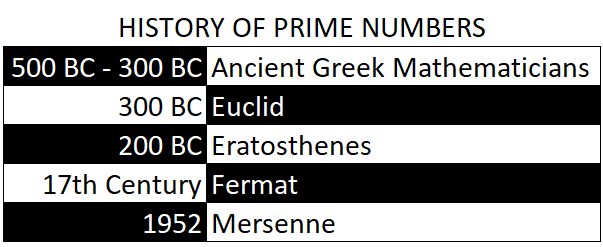Properties, twin primes and co-primes

### Twin Prime Numbers

Prime Numbers that differ by 2 are known as Twin Prime Numbers
For example, a list of Prime Numbers between 1 to 10 is displayed below,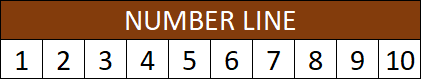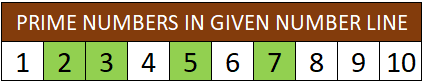Let us consider the first two Prime Numbers in the above number line.
It is 2 and 3.
The difference between 2 and 3 is 1 (3 - 2 = 1)
Hence, 2 and 3 are not Twin Prime Numbers

Let us consider the second pair of Prime Numbers in the given number line.
It is 3 and 5.
The difference between 3 and 5 is 2 (5 - 3 = 2)
Hence, 3 and 5 are Twin Prime Numbers

Similarly let us try with the third pair of Prime Numbers in the given number line.
It is 5 and 7.
The difference between 5 and 7 is 2 (7 - 3 = 2)
Hence, 5 and 7 are Twin Prime Numbers

As the numbers keep increasing in the number line, Prime Numbers become less frequent and Twin Prime Numbers become rare to be found in the series.

### Co-Prime Numbers

Co-prime numbers are a set of numbers which have only 1 as their common factor. Co means two, and hence in order to identify Co-Prime Numbers we will be in need of two numbers.
For Example
Let us consider the pair of numbers 18 and 35.
18 can be broken or in other words can be written as product of two numbers as below,
1 x 18 = 18
2 x 9   = 18
3 x 6   = 18
6 x 3   = 18
9 x 2   = 18
18 x 1 = 18
From the above list of product of two numbers, the Factors of 18 are 1, 2, 3, 6, 9 and 18

Let us work out the same for number 35, which is the pairing number to 18 in the given statement.
35 can be broken or in other words can be written as product of two numbers as below,
1 x 35 = 35
5 x 7   = 35
7 x 5   = 35
35 x 1 = 35
From the above list of product of two numbers, the Factors of 35 are 1, 5, 7 and 35

Comparing the factors of 18 and 35, 1 appears to be the only common factor, thus satisfying the property of a pair of numbers to be termed as Co-Prime Numbers.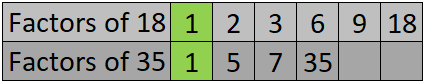Let us work out for another set of numbers to identify the difference between Co-Prime Numbers and Non Co-Prime Numbers.
In this case, let us take 18 and 19
The outcome is as shown in the below image,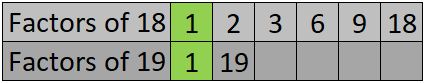As the only common Factor for numbers 18 and 19 is 1, Numbers 18 and 19 can be termed as Co-Prime Numbers.

Another Example,
In this case, let us take 18 and 20
Though 1 is a common Factor for 18 and 20, yet there is another common Factor in the list which is number 2.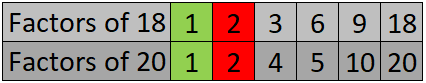The Property of a Co-Prime Number is that it should have only 1 as the Common Factor. In this case, we also have 2 along with 1 as Common Factor and hence numbers 18 and 20 are not Co-Prime in nature.

Interesting fact about 1 is that it is a Co-Prime Number for all the numbers.

## Sieve of Eratosthenes

It is an algorithm to find all given numbers up to a limit.

The algorithm to find prime numbers between 1- 100 proceeds like this.

### Step 1

Make a table 0f 10 rows and 10 columns starting with 1 continuing until 100, as displayed in the below image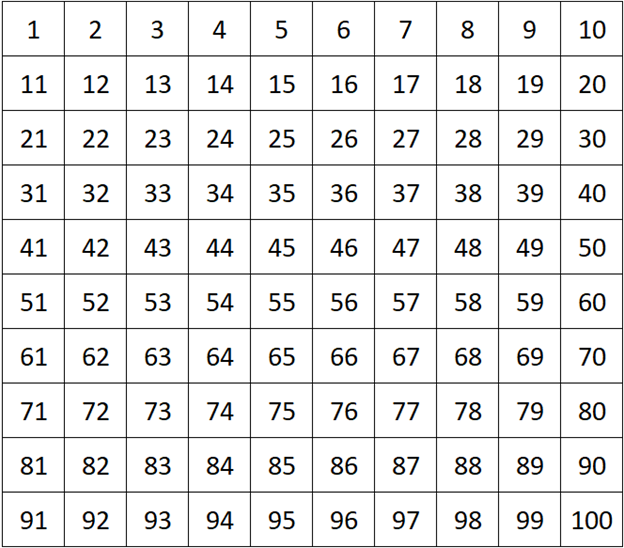### Step 2

Based on the above illustrations it is evident that 2 is a prime number.

Mark 2 in the table as a prime number, by circling it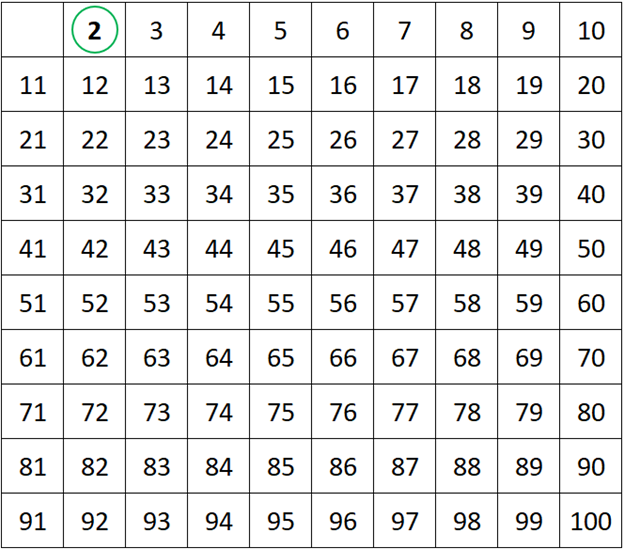### Step 3

Mark all the multiples of 2, this will help eliminate the composite numbers from the list thus highlighting only the primes step by step.

The multiple of 2 are marked in grey, to emphasize that the numbers which are not prime in nature are ignored.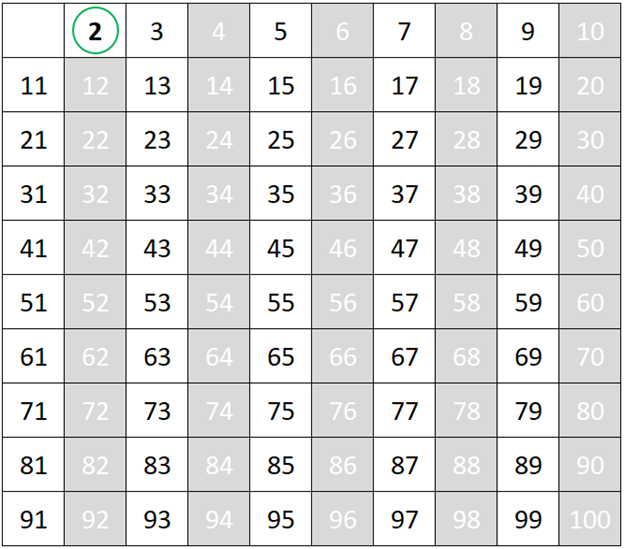### Step 4

Now, find the smallest number in the list greater than 2 that is not marked, which will be the next prime number in the list. In this case, it is 3. Circle 3, and repeat step 3 to eliminate the multiples of 3.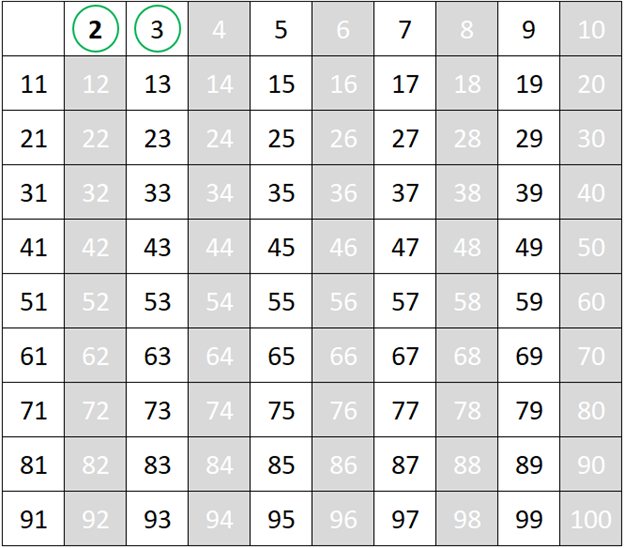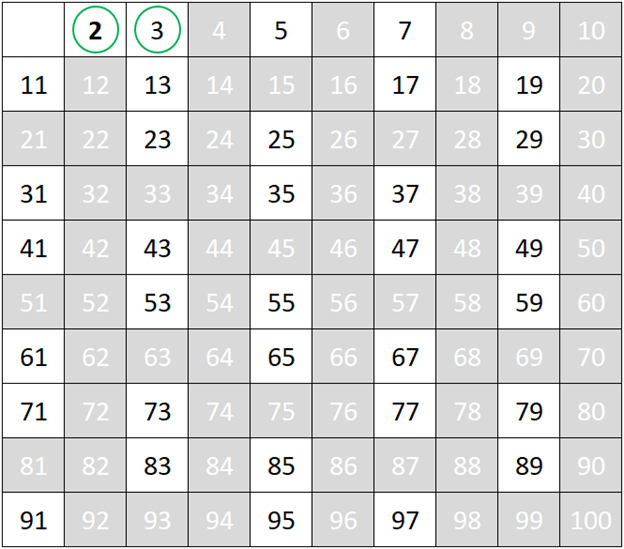### Step 5

Repeat Step 4 to find the next smallest number in the list which will be the next prime number, followed by will elimination of multiples of the prime number as per step 3

In this case, the next smallest number in the list is 5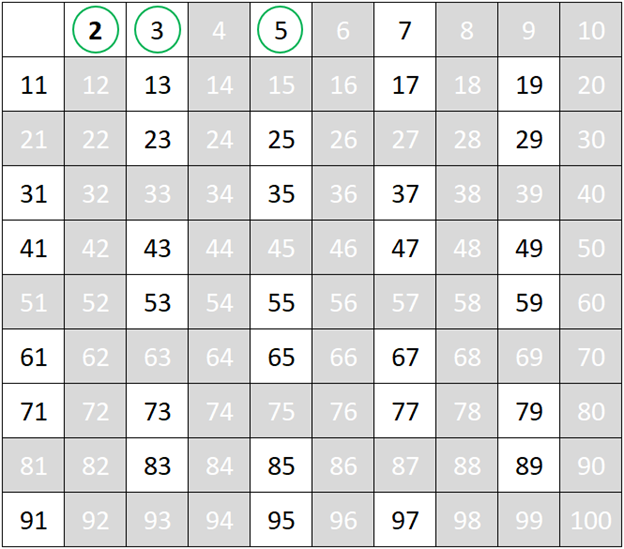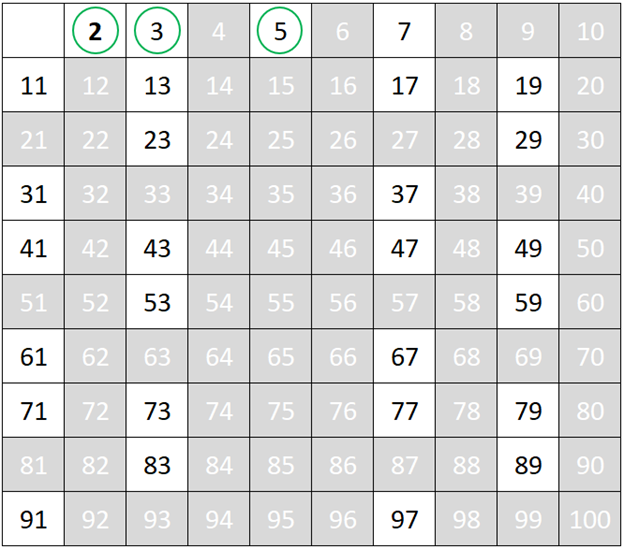### Step 6

Repeat Step 4 to find the next smallest number in the list which will be the next prime number, followed by will elimination of multiples of the prime number as per step 3

In this case, the next smallest number in the list is 7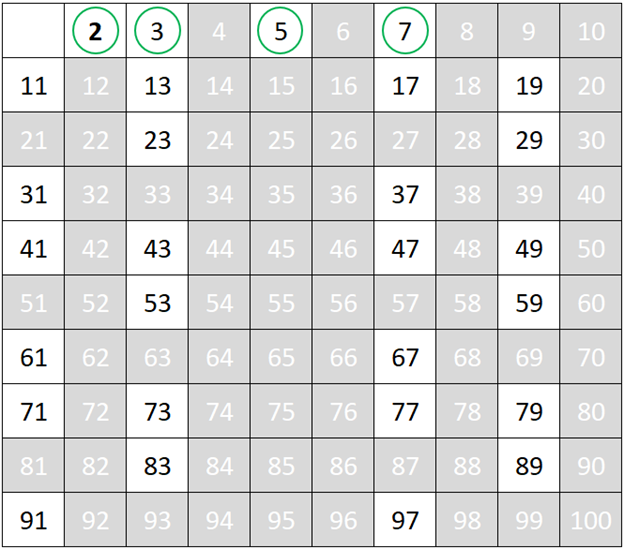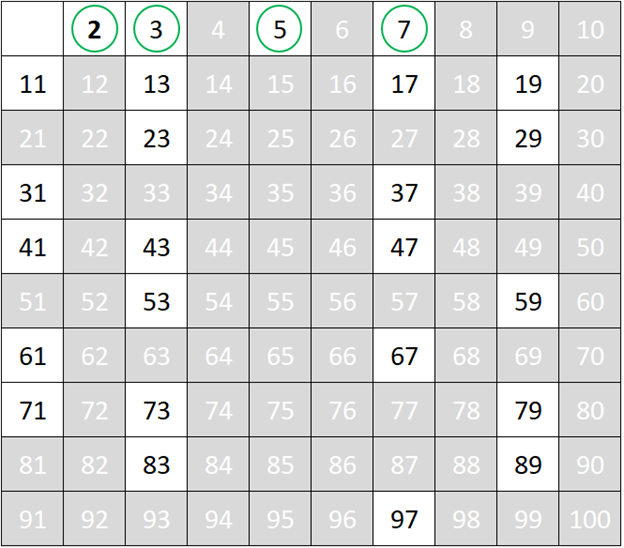### Step 7

Repeat Step 4 to find the next smallest number in the list which will be the next prime number, followed by will elimination of multiples of the prime number as per step 3

In this case, the next smallest number in the list is 11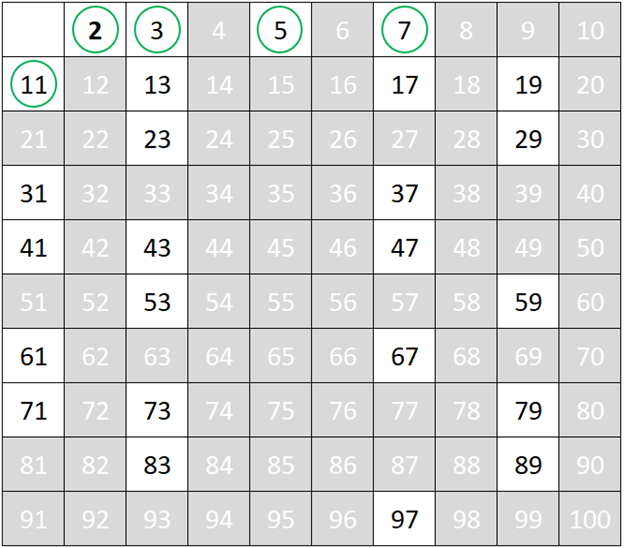### Step 8

Repeat Step 4 to find the next smallest number in the list which will be the next prime number, followed by will elimination of multiples of the prime number as per step 3

In this case, the next smallest number in the list is 13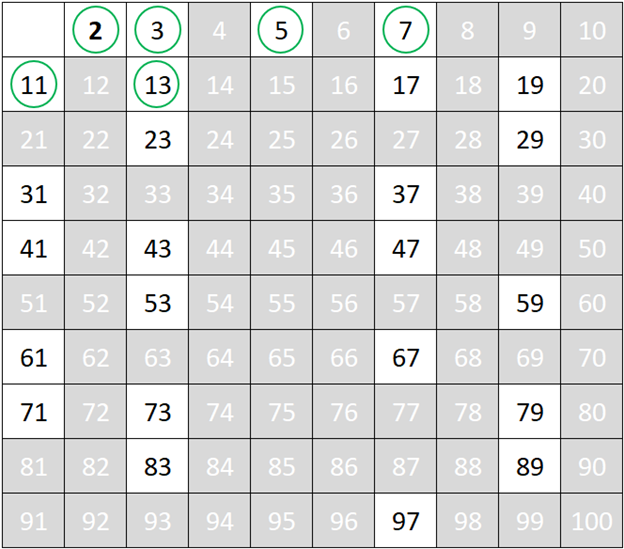### Step 9

Repeat Step 4 to find the next smallest number in the list which will be the next prime number, followed by will elimination of multiples of the prime number as per step 3

In this case, the next smallest number in the list is 17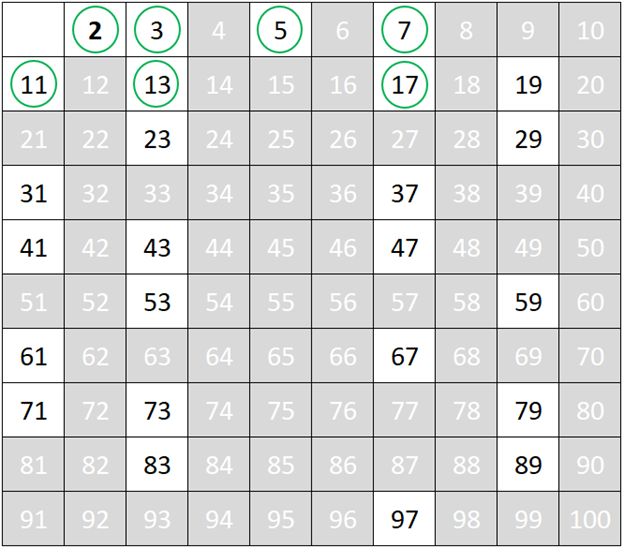### Step 10

Repeat Step 4 to find the next smallest number in the list which will be the next prime number, followed by will elimination of multiples of the prime number as per step 3

In this case, the next smallest number in the list is 19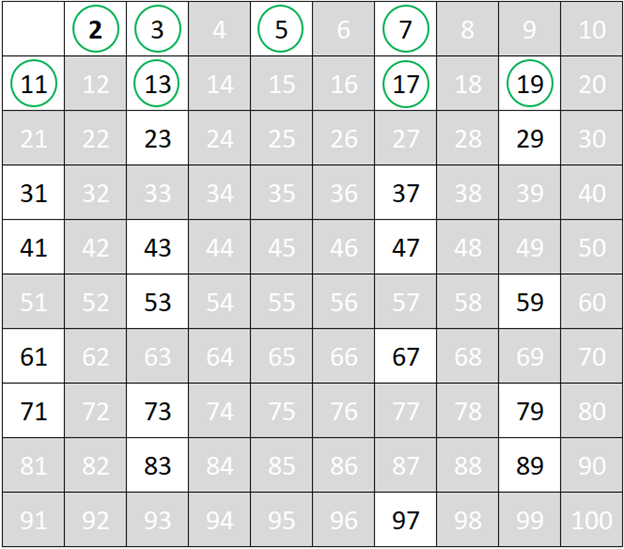### Step 11

Repeat Step 4 to find the next smallest number in the list which will be the next prime number, followed by will elimination of multiples of the prime number as per step 3

In this case, the next smallest number in the list is 23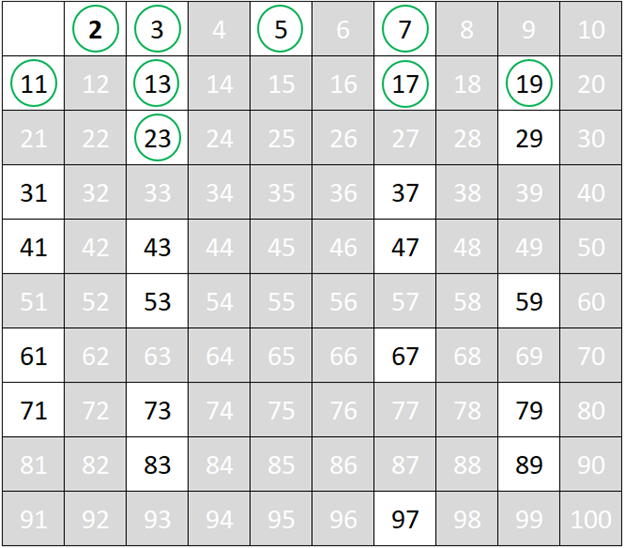### Step 12

Repeat Step 4 to find the next smallest number in the list which will be the next prime number, followed by will elimination of multiples of the prime number as per step 3

In this case, the next smallest number in the list is 29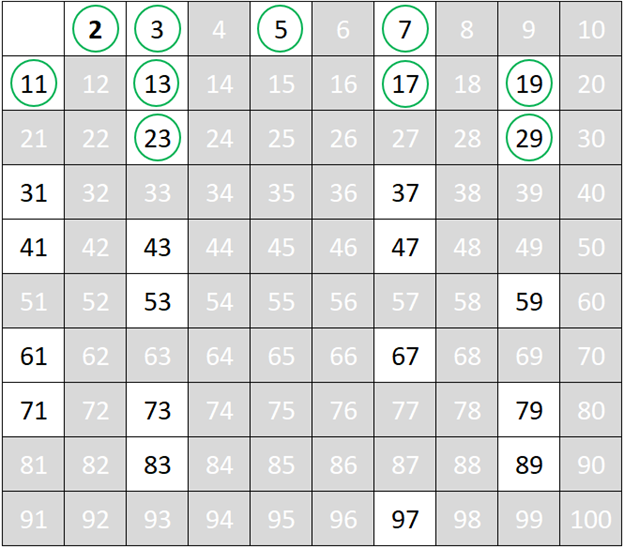### Step 13

Repeat Step 4 to find the next smallest number in the list which will be the next prime number, followed by will elimination of multiples of the prime number as per step 3

In this case, the next smallest number in the list is 31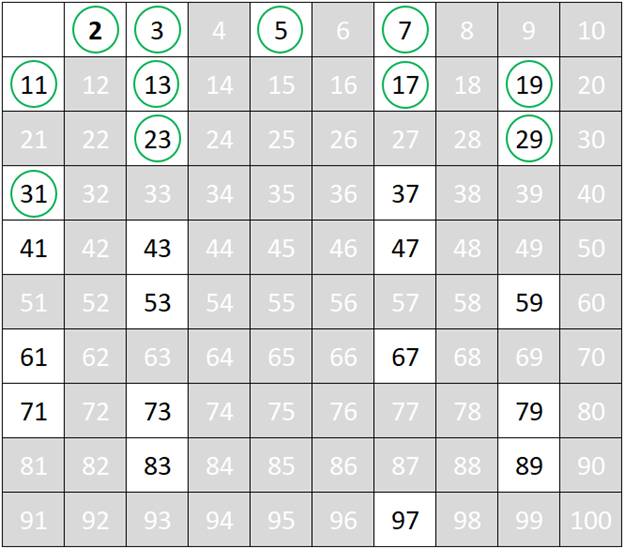### Step 14

Repeat Step 4 to find the next smallest number in the list which will be the next prime number, followed by will elimination of multiples of the prime number as per step 3

In this case, the next smallest number in the list is 37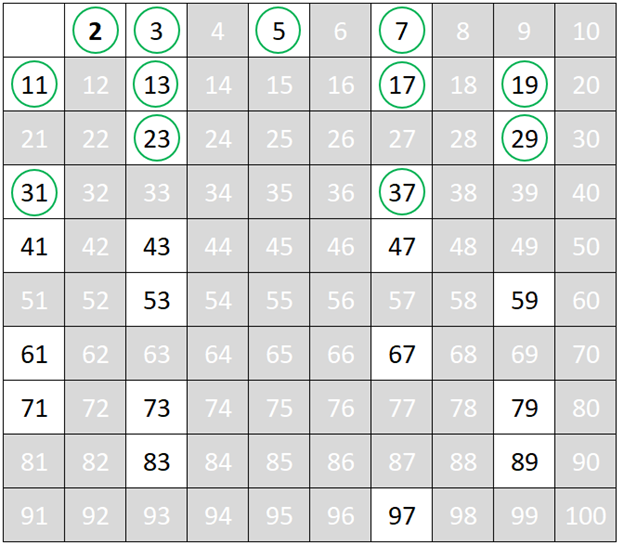### Step 15

Repeat Step 4 to find the next smallest number in the list which will be the next prime number, followed by will elimination of multiples of the prime number as per step 3

In this case, the next smallest number in the list is 41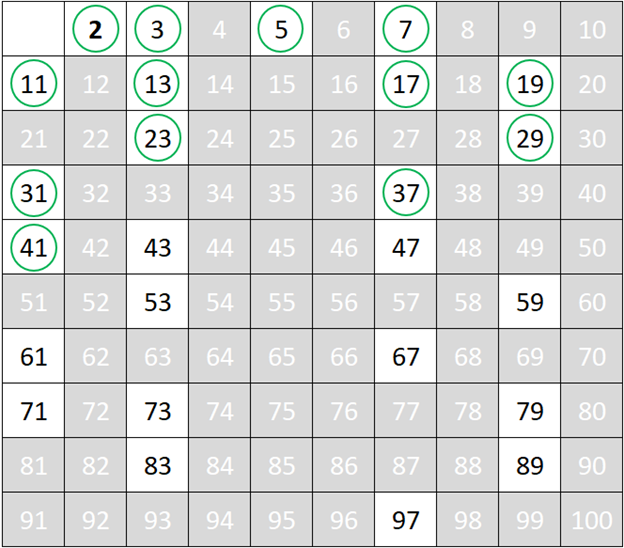### Step 16

Repeat Step 4 to find the next smallest number in the list which will be the next prime number, followed by will elimination of multiples of the prime number as per step 3

In this case, the next smallest number in the list is 43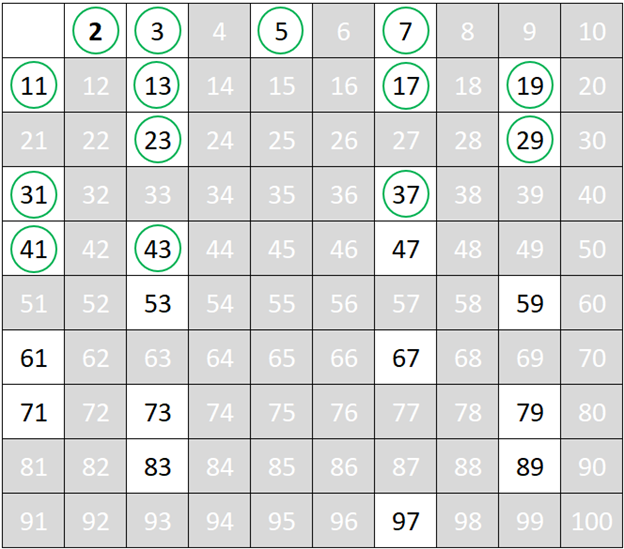### Step 17

Repeat Step 4 to find the next smallest number in the list which will be the next prime number, followed by will elimination of multiples of the prime number as per step 3

In this case, the next smallest number in the list is 47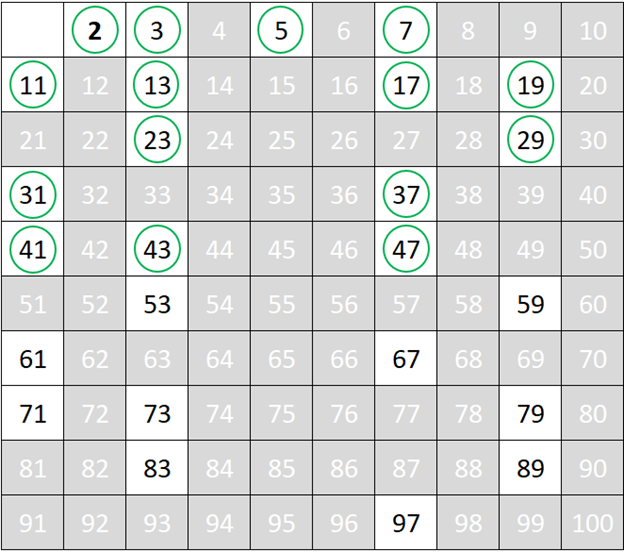### Step 18

Repeat Step 4 to find the next smallest number in the list which will be the next prime number.

In this case, the next smallest number in the list is 53

The elimination of multiples from this prime number may not be required as the table does not hold numbers greater than 100.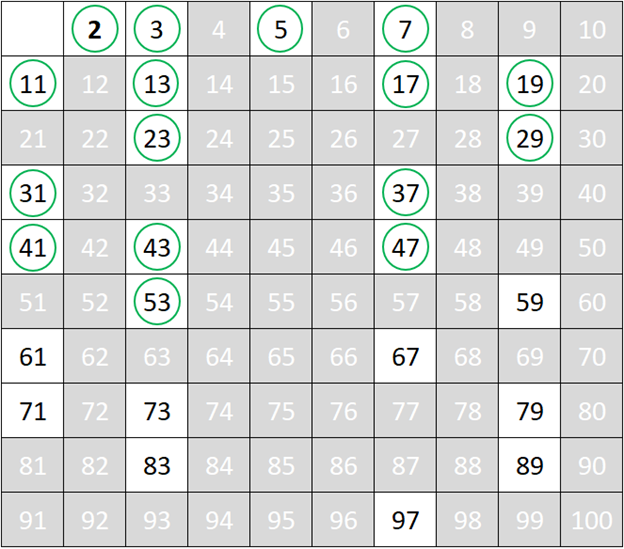### Step 19

Repeat Step 4 to find the next smallest number in the list which will be the next prime number,

In this case, the next smallest number in the list is 59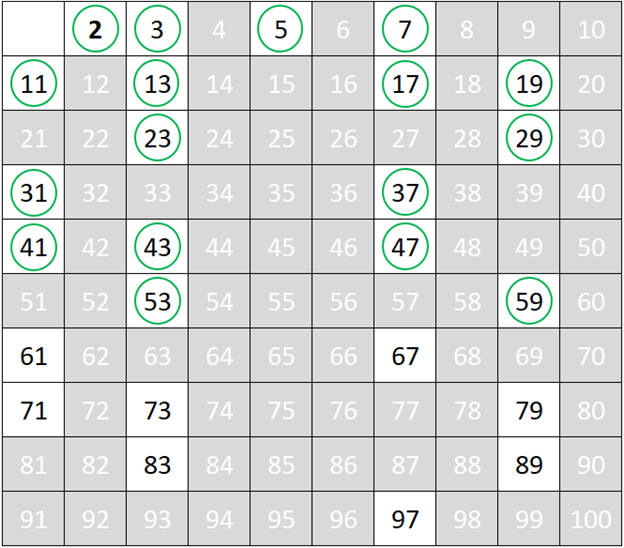### Step 20

Repeat Step 4 to find the next smallest number in the list which will be the next prime number,

In this case, the next smallest number in the list is 61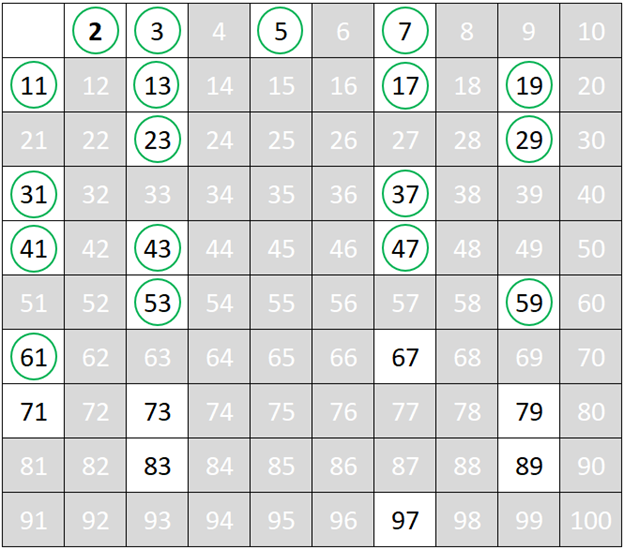### Step 21

Repeat Step 4 to find the next smallest number in the list which will be the next prime number,

In this case, the next smallest number in the list is 67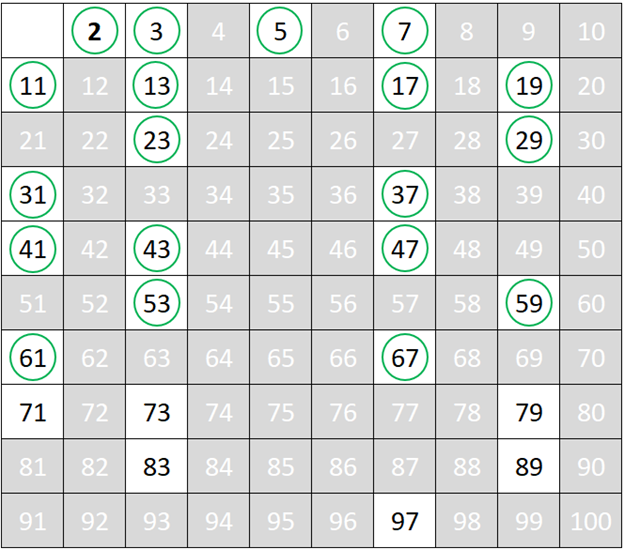### Step 22

Repeat Step 4 to find the next smallest number in the list which will be the next prime number,

In this case, the next smallest number in the list is 71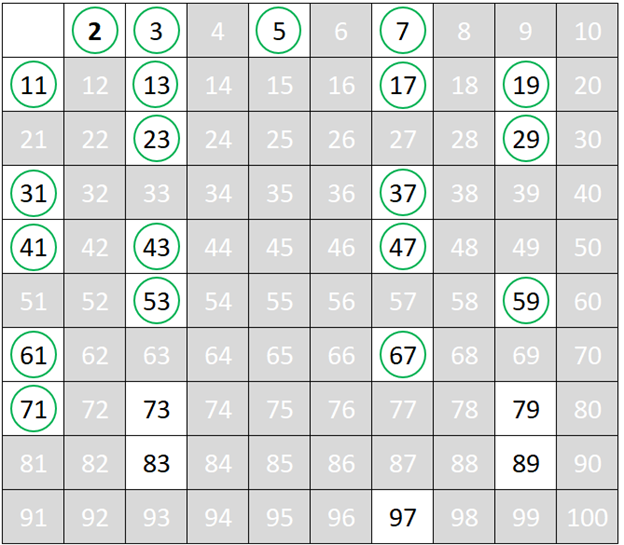### Step 23

Repeat Step 4 to find the next smallest number in the list which will be the next prime number,

In this case, the next smallest number in the list is 73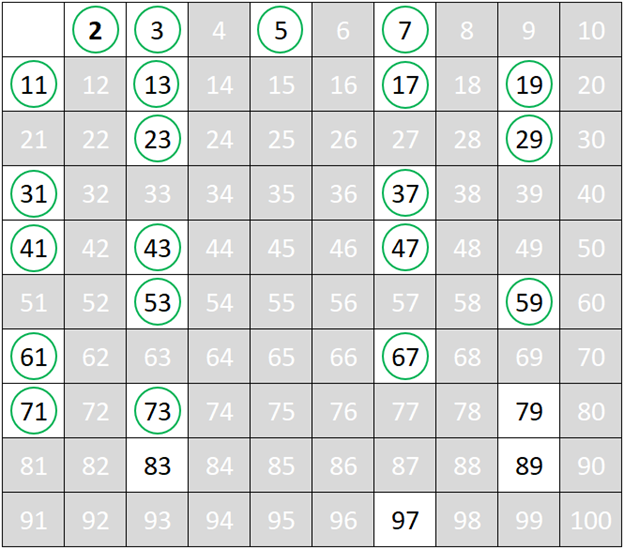### Step 24

Repeat Step 4 to find the next smallest number in the list which will be the next prime number,

In this case, the next smallest number in the list is 79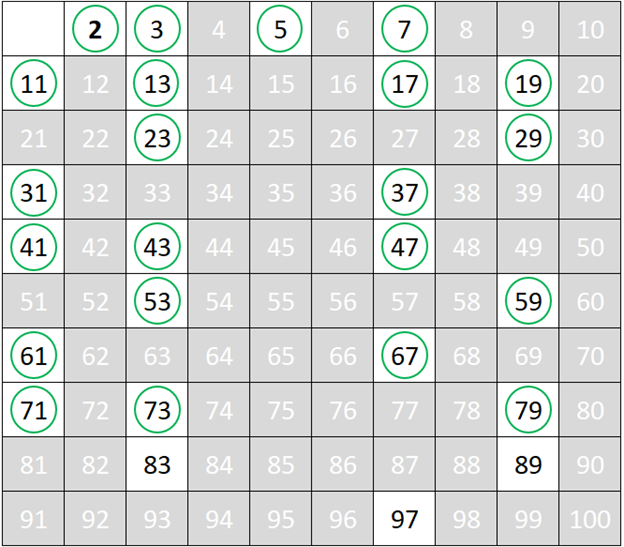### Step 25

Repeat Step 4 to find the next smallest number in the list which will be the next prime number,

In this case, the next smallest number in the list is 83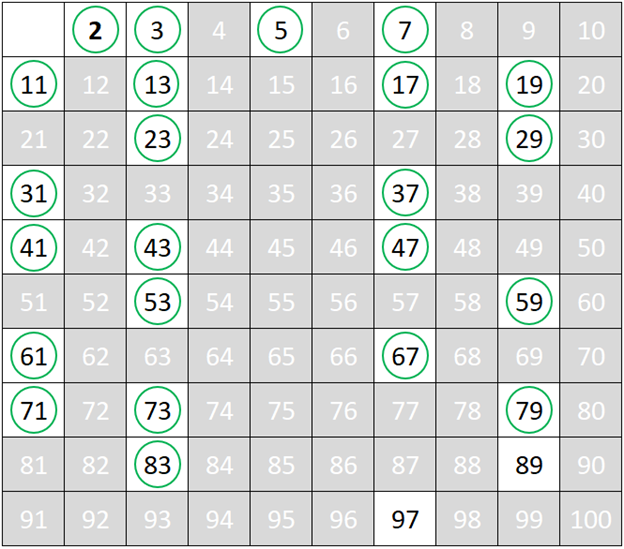### Step 26

Repeat Step 4 to find the next smallest number in the list which will be the next prime number,

In this case, the next smallest number in the list is 89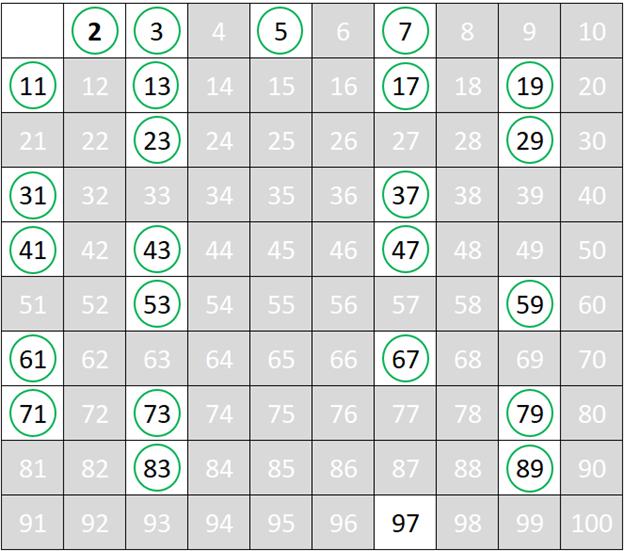### Step 27

Repeat Step 4 to find the next smallest number in the list which will be the next prime number,

In this case, the next smallest number in the list is 97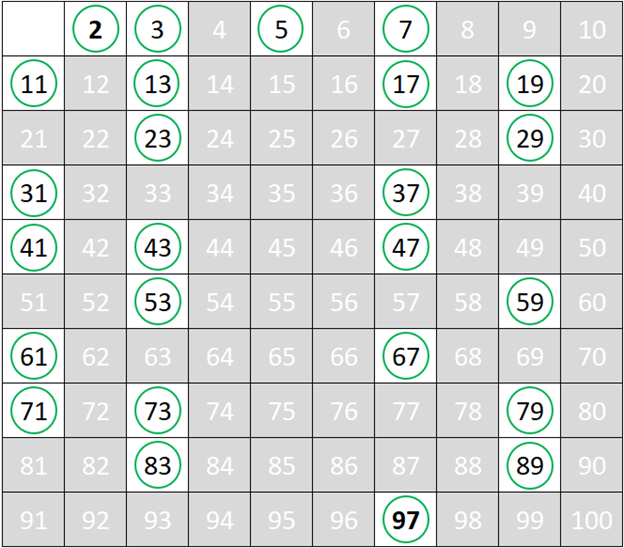Based on the Sieve of Eratosthenes, the numbers that are classified as prime falling between 1 – 100 are, 2 3 5 7 11 13 17 19 23 29 31 37 41 43 47 53 59 61 67 71 73 79 83 89 97

## What are co-prime numbers?

Pair of Numbers that have their greatest common divisor i.e. GCD is unity, which means that the only number that can divide each of them is 1.

## How to find prime numbers?

Prime Numbers can be found by using the Sieve of Eratosthenes.

## How many prime numbers are from 1-100?

There are 25 prime numbers from 1-100.

## What are twin prime numbers?

The pair of primes that differ by an amount of 2 are known as twin prime numbers.

## What is the sieve of Eratosthenes?

It is an algorithm to find all given numbers up to a limit.

GIVE YOUR CHILD THE CUEMATH EDGE
Access Personalised Math learning through interactive worksheets, gamified concepts and grade-wise courses